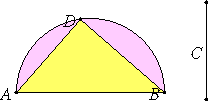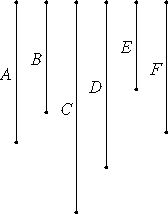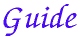# Lemma.

Given two unequal straight lines, to find by what square the square on the greater is greater than the square on the less.

Let AB and C be the given two unequal straight lines, and let AB be the greater of them.

It is required to find by what square the square on AB is greater than the square on C.IV.1

Describe the semicircle ADK on AB, fit AD into it equal to C, and join DB.

It is then clear that the angle ADB is right, and that the square on AB is greater than the square on AD, that is, C, by the square on DB.

Similarly also, if two straight lines are given, then the straight line the square on which equals the sum of the squares on them is found in this manner.

Let AD and DB be the given two straight lines, and let it be required to find the straight line the square on which equals the sum of the squares on them.

Place them so as to contain a right angle ADB, and join AB.

I.47

It is again manifest that the straight line the square on which equals the sum of the squares on AD and DB is AB.

Q.E.D.

# Proposition 14

If four straight lines are proportional, and the square on the first is greater than the square on the second by the square on a straight line commensurable with the first, then the square on the third is also greater than the square on the fourth by the square on a third line commensurable with the third. And, if the square on the first is greater than the square on the second by the square on a straight line incommensurable with the first, then the square on the third is also greater than the square on the fourth by the square on a third line incommensurable with the third.
Lemma

Let A, B, C, and D be four straight lines in proportion, so that A is to B as C is to D, and let the square on A be greater than the square on B by the square on E, and let the square on C be greater than the square on D by the square on F.

I say that, if A is commensurable with E, then C is also commensurable with F, and, if A is incommensurable with E, then C is also incommensurable with F.VI.22

Since A is to B as C is to D, therefore the square on A is to the square on B as the square on C is to the square on D.

But the sum of the squares on E and B equals the square on A, and the sum of the squares on D and F equals the square on C. Therefore the sum of the squares on E and B is to the square on B as the sum of the squares on D and F is to the square on D.

Therefore, taken separately, the square on E is to the square on B as the square on F is to the square on D. Therefore E is to B as F is to D. Therefore, inversely, B is to E as D is to F.

V.22

But A is to B as C is to D, therefore, ex aequali, A is to E as C is to F.

X.11

Therefore, if A is commensurable with E, then C is also commensurable with F, but if A is incommensurable with E, then C is also incommensurable with F.

Therefore, if four straight lines are proportional, and the square on the first is greater than the square on the second by the square on a straight line commensurable with the first, then the square on the third is also greater than the square on the fourth by the square on a third line commensurable with the third. And, if the square on the first is greater than the square on the second by the square on a straight line incommensurable with the first, then the square on the third is also greater than the square on the fourth by the square on a third line incommensurable with the third.

Q.E.D.

###A little modern algebra clarifies the situation. We assume A : B = C : D. Then if √(A2 – B2) : A is a numeric ratio, then so is √(C2 - D2) : C. It’s simply because √(A2 – B2) : A = √(C2 – D2) : C.

The lemma is the same as the lemma for proposition XI.23.

The proposition is used in several propositions in Book X starting with X.31.